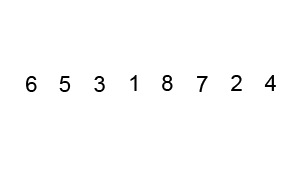# C Bubble Sort

Summary: this tutorial explains how the bubble sort algorithm works and shows you how to implement the bubble sort in C.

##Introduction to the bubble sort algorithm

Bubble sort is a simple sorting algorithm. The bubble sort works by arranging adjacent elements repeatedly if they are not in the correct order. When no exchange is needed, the list is sorted.

By doing this, the smaller element bubble to the top, that why this sorting technique is called bubble sort.

Bubble sort is the simplest sorting algorithm is are easy to understand and quick to implement. However, in practice, it is not recommended.

The complexity of the bubble sort algorithm is O(n2). Its efficiency decreases dramatically when the number of elements in the unsorted list increases.

Among the  O(n2) sorting algorithms, the insertion sort is more efficient than the bubble sort.

## C bubble sort implementation

The following is the C program for bubble sorting demonstration. We use the bubble sort technique to sort an array of integers.

In this tutorial, you have learned about the bubble sort algorithm and how to implement it in C.